Welcome to the sixth installment in my series Getting Started with Python Integration to SAS Viya. In previous posts, I discussed how to connect to the CAS serverhow to execute CAS actions, and how to work with the results. Now it's time to generate simple descriptive statistics of a CAS table.

Let's begin by confirming the cars table is loaded into memory. With a connection to CAS established, execute the read_csv SWAT method to load the cars.csv file into the CAS server. Then execute tableInfo action to view available in-memory tables.

```# Load demo data into the CAS server conn.read_csv('https://support.sas.com/documentation/onlinedoc/viya/exampledatasets/cars.csv', casout = {'name':'cars', 'caslib':'casuser'}) conn.tableinfo(caslib="casuser")```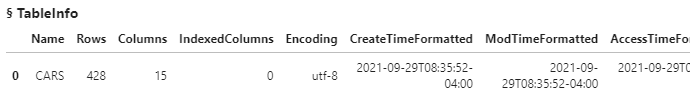The results show the cars table is loaded into memory and available for processing. Next, reference the cars table in the variable tbl. Then use the print function to show the value of the variable.

```tbl = conn.CASTable('cars', caslib='casuser') print(tbl) CASTable('cars', caslib='casuser')```

The results show that the tbl variable references the cars table in the CAS server.

## Preview the CAS Table

First things first. Remember, the SWAT package blends the world of Pandas and CAS into one. So you can begin with the traditional head method to preview the CAS table.

`tbl.head()`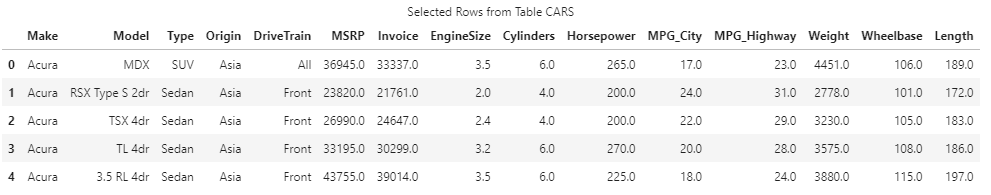The SWAT head method returns five rows from the CAS server to the client as expected.

## The Describe Method

Next, let's retrieve descriptive statistics of all numeric columns by using the familiar describe method on the CAS table.

`tbl.describe()`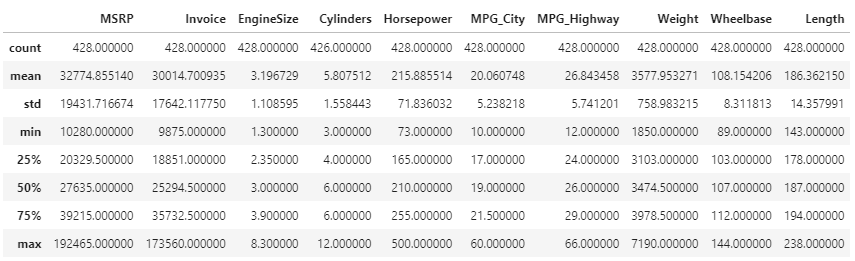The SWAT describe method returns the same descriptive statistics as the Pandas describe method. The only difference is that the SWAT version uses the CAS API to convert the describe method into CAS actions behind the scenes to process the data on the distributed CAS server. CAS processes the data and returns summarized results back to the client as a SASDataFrame, which is a subclass of the Pandas DataFrame. You can now work with the results as you would a Pandas DataFrame.

## Summary CAS Action

Instead of using the familiar describe method, let's use a CAS action to do something similar. Here I'll use the summary CAS action.

`tbl.summary()`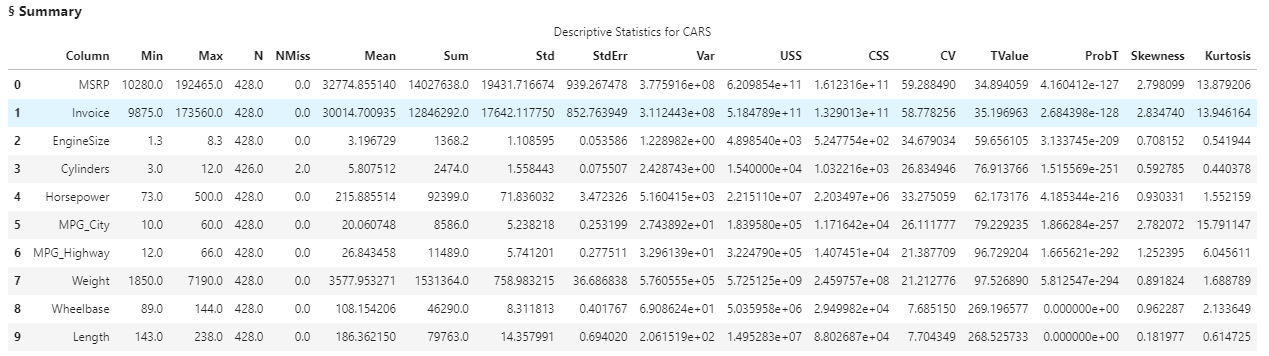The results of the summary action return a CASResults object (Python dictionary) to the client. The CASResults object contains a single key named Summary with a SASDataFrame as the value. The SASDataFrame shows a variety of descriptive statistics.  While the summary action does not return exactly the same statistics as the describe method, it can provide additional insights into your data.

What if we don't want all the statistics for all of the data?

## Selecting Columns and Summary Statistics with the Summary Action

Let's add additional parameters to the summary action. I'll add the inputs parameter to specify the columns to analyze in the CAS server.

`tbl.summary(inputs = ['MPG_City','MPG_Highway'])`The results show only the MPG_City and MPG_Highway columns were analyzed.

Next, I'll use the subSet parameter to specify the summary statistics to produce. Here I'll obtain the MEAN, MIN and MAX.

```tbl.summary(inputs = ['MPG_City','MPG_Highway'], subSet = ['mean','min','max'])```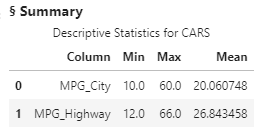The results processed only the MPG_City and MPG_Highway columns, and returned only the specified summary statistics to the client.

## Creating a Calculated Column

Lastly, let's create a calculated column within the summary action. There are a variety of ways to do this. I like to add it as a parameter to the CASTable object. You can do that by specifying the tbl object, then computedVarsProgram parameter. Within computedVarsProgram you can use SAS assignment statements with most SAS functions. Here we will create a new column name MPG_Avg that takes the mean of MPG_City and MPG_Highway. Lastly, add the new column to the inputs parameter.

```tbl.computedVarsProgram = 'MPG_Avg = mean(MPG_City, MPG_Highway);' tbl.summary(inputs = ['MPG_City','MPG_Highway', 'MPG_Avg'], subSet = ['mean','min','max'])```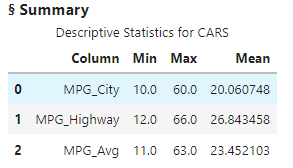In the results I see the calculated column and requested summary statistics.

## Summary

The SWAT package blends the world of Pandas and CAS. You can use many of the familiar Pandas methods within the SWAT package, or the flexible, highly optimized CAS actions like summary to easily obtain summary statistics of your data in the massively parallel processing CAS engine.